## Saturday, 24 December 2011

### Mathematical Induction and its consequences -- Finale

P.S. In before this starts. I decided to insert the method of induction into my summarizing work, Basic Albegraic Skills.

"Numbers" in history have different meanings. In the past there'are only natrual numbers by counting, next there was negative numbers due to the concept of debt. Rational numbers seems to fill up the real continum but Pythagoreans has found irrational numbers. Finally the concept of real number continum is developed by Cantor and complex numbers appeared as powerful calculation tool.

Mathematical induction, processing natrual numbers, seems too "old fashioned" because there're far many problems concerning somewhere beyond natrual numbers, and we need different approaches. If MI can be extended over the reals, it's just fantastic. We will illustrate this by two examples: function equation and bionomial theorem over the reals. Before that, we will look at more different MI problems on A-level exam past papers.

Theorem. (Generalization of M.I.)
If (a) P(a) and P(b) is true implies that P(a+b), P(|a-b|) is true,
b) Exist least r that P(r) is true,
then P(k) is true is equivalent to k is a multiple of r.

6. N, Z, Q, R --- extented conclusion derived from MI

Example 16. f(x) is a everywhere continuous and differentiable function satisfying:
1)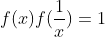for non-zero x;
2) Additive, i.e.,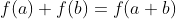;
3) f(1)=1.
Show that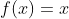.

Step 1: N
For the proprosition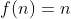: assume n=k is true, and for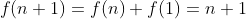is also true.

Step 2: Z, therefore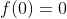.
Next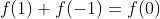, therefore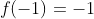.
Now set the proposition "f(-n)=-n" and finish the induction.

Another approach is that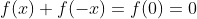, therefore f is even and it's true over integers.

Step 3: Q
We use a double MI:
Theorem. The proposition P(a,b) is true for all a,b if:
1) P(1,1) is true.
2) If P(a,b) is true, then P(a,b+1) is true.
3) If P(a,b) is true, then P(a+1,b) is true.
This is quite powerful for rational numbers.
Here we use another approach of double MI that "For every b, P(a,b) implies P(a+1,b) is true".
For any given n,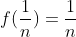by property 1, and do induction so that "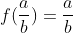for every b. The method is similar as step 1 and 2. This is true for all rational numbers.

Step 4: R
We know that irrational numbers can always be traced by a series of rational numbers.
Lemma. Every irrational numbers can be written in forms of sum of rational numbers.
Then if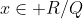and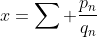, then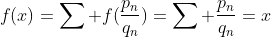.

P.S. Yet there's a simple method to do this question. Since the function is additive, we can show that the function has a constant slope, i.e., y' = m, so y = mx+c. Considering f(0)=0 and f(1)=1, we have f(x)=x.
Example 17. Extension of the binomial theorem.

Lemma. Vandermonde's Theorem: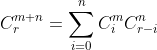The proof is trivial when you expand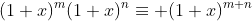and compare the coefficients of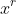.

Now consider the function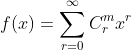where f converges when |x| < 1. Now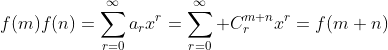(This can be done by direct expanding.)

Now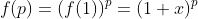so that it's also valid for real p.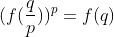, so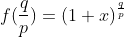in which the positive root is taken due to positive sum is given.

For negative numbers, we have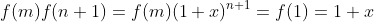, then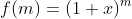.

For real numbers, we know that f is everywhere continous and differentiable in the interval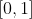, therefore we can also put a irrational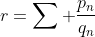that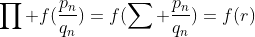.

By comparing the coefficients we have the binomial coefficients over real numbers: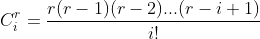and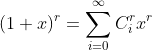. When r is integer,for r < n so that the sum is finite. These two results are quite powerful and the following problems can be solved. Exercise.
1) Show that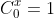for all real x.
2) Show that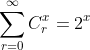is valid over real x by:
a) binomial substitution
b) the NZQR approach.
c) Compute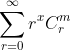where m and x is a real number.
3) Show the following identities:
a)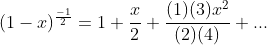b)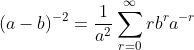where. Is it valid when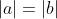?
4) (2004 Pure I 5 modified) For real x, |a| < 1, show that: a),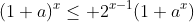, hence show that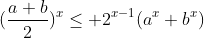Read the rest of this passage:

Part I
Part II
Part III